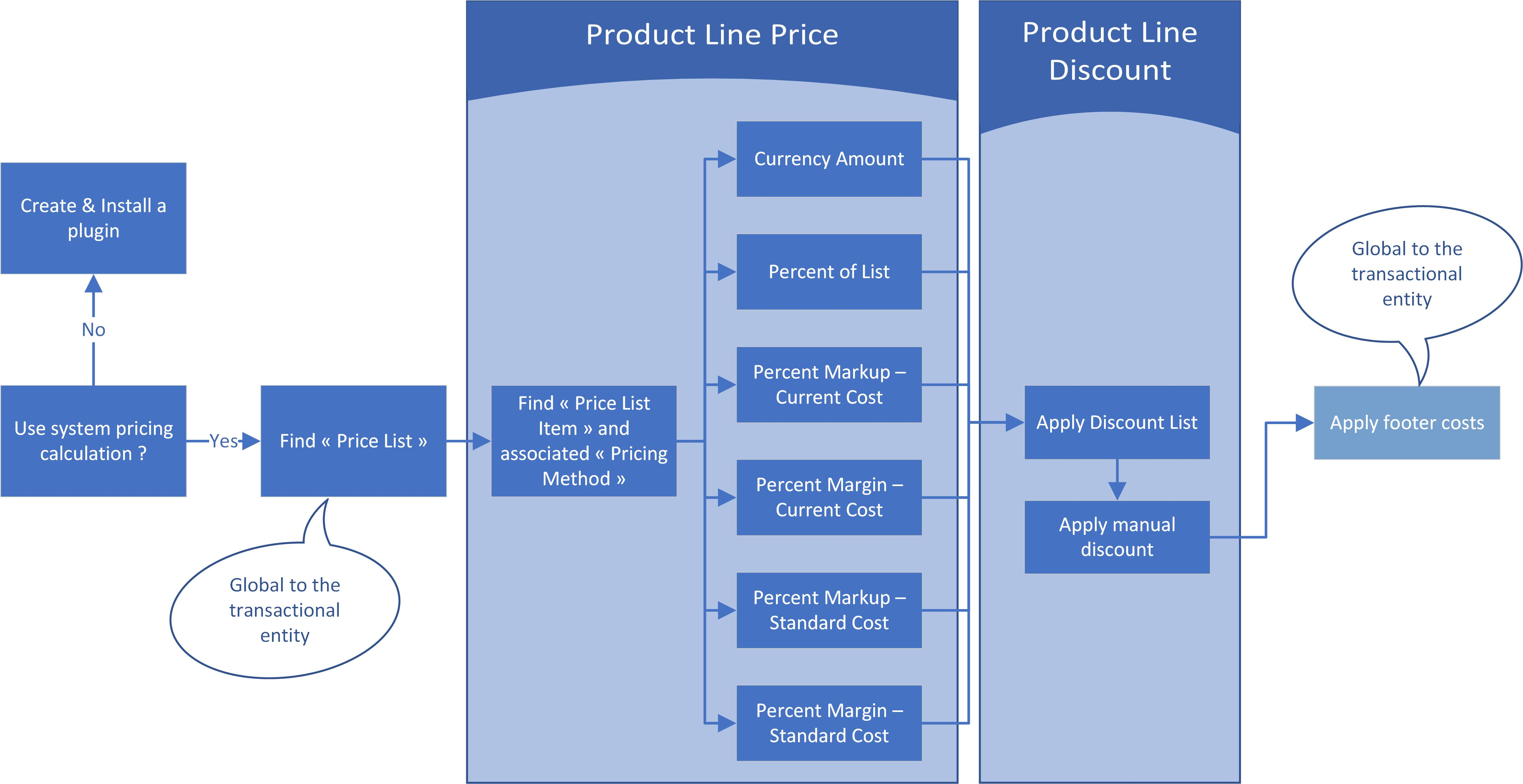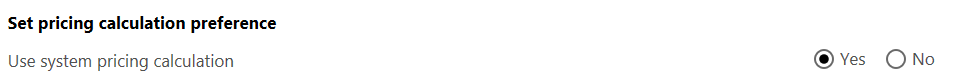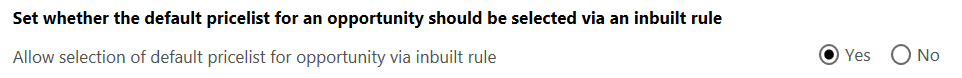# Dynamics 365 : Product Prices

Determining the price calculation rules of a product in an opportunity or transactional entity (quote, order or invoice) may be quite complex, due to the multiple combinations available. This complexity gives the freedom to comply to various business needs.

I created the following schema to summurize these options, and will explain them in more details. Most of the options relate to the « Product Line », some are global to the whole entity.# Use system pricing calculation

First of all, we need to check if system pricing calculation rules are applied (which is the cas by default), in system settings (in the Sales tab) :If it is not the case, a plug-in should be created and registered (will be explained in a future post).

# Find « Price List »

Assuming we use System pricing calculation, you need to choose an applicable Price List for your whole entity. Take care that a given Price List relates to unique currency : it must be the same than that of your entity. Moreover, you will need to have « Price List Item » for any product added in the lines.
Default value : if there exists a « Sales Territory » assigned to you, and that Sales Territory is attached to one (and only one) Price List, that Price List will be selected by default, unless you turn that functionnality off in system settings :# Find « Price List Item » and associated « Pricing Method »

Once you have choosen a « Price List », you need to add products to your transaction entity. The price that will be applied is retrieved from the « Price List », if a releavant « Price List Item » exists : the same Product with the same Unit as selected in the transactional entity.
The Price List Item defines the « Pricing Method » :

• Currency Amount : a fixed amount
Percent of List : the « List Price » is defined on the Product : Percentage * List Price
• Percent markup – current cost : the « Current Cost » is defined on the Product. Percent Markup is Current Cost * ( 100 + Percentage) / 100
• Percent margin – current cost : the « Current Cost » is defined on the Product. Percent Margin is Current Cost + (Current Cost * Percentage) / (100 – Percentage))
• Percent markup – standard cost : the « Standard Cost » is defined on the Product. Percent Markup is Standard Cost * ( 100 + Percentage) / 100
• Percent margin – standard cost : the « Standard Cost » is defined on the Product. Percent Margin is Standard Cost + (Standard Cost * Percentage) / (100 – Percentage))

Attention : by default, the fields « List Price », « Standard Cost » and « Current Cost » may not be displayed on the Product form. It is easy to customize and add these.
What are the meaning of these fields? You can assign the meaning you want, but usually :

• List Price : the official sales Price from an internal « Sales List »
• Current Cost : the current (temporary) price of a Product – maybe the Price payed to a provider
• Standard Cost : the typical price of a Product
• « Percent Margin » is greater than « Percent Markup ».

Percent Margin means : the Percentage of the calculated price that I want as a margin
Percent Markup means : the Percentage of the initial Price that I want to add.
There are some more options in the « Price List Item » that will fine-tune the calculated price of a Product from a Price List :

• Rounding Policy : None, Up, Down, Nearest
• Rounding Option (unavailable for the « None » Rounding Policy) :
• « Ends In » : forces the Price to end with for example with « .99 », so that a calculated price of 50.14 may be automatically rounded to 49.99
• « Multiple of » : forces to Price to be a multiple of a certain amount – for example, a calculated price of 50.14 may be automatically rounded to 50.10 if the « Rounding Amount » is « 0.10 »
• Rounding Amount : the amount used by « Rounding Option »

For example, with a cost of 50 and a Percentage of 10 :

• The Percent Margin is 50 + (50 * 10) / (100 – 10) = 55,56 => my Margin is 5,56 which is 10% of 55,56
• The Percent Markup is 50 * (100 + 10) / 100 = 55,0 => my Markup is 5 which is 10% of 50

# Apply Discount List

Configuration : You can create « Discount Lists ». A Discount List is basicaly an entity specifying the discounts applied depending in quantity (whether an amount, or a percentage) on any product. Then, you attach this Discount List to a « Price List Item » in your Product Catalog configuration.
The discount will be automatically applied on the line.

# Apply Manual Discount

You can manually add a absolute discount on the Product Line Item.

Apply footer costs